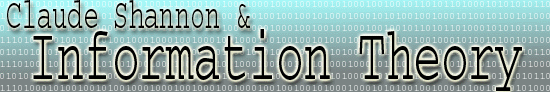Fano Codes

Claude Shannon R.M. Fano independently came up with this method of coding that is always the most efficient whenever it is possible. For an example, take as the set of possible symbols the four bases found in DNA, adenine, thymine, cytosine, and guanine, as the set of elements. There is no coincidence that this was chosen as the example, Information Theory is highly applicable to genetics! We shall abbreviate these as A, T, C, and G, respectively. The probabilities of each are as follows: adenine - 0.5, thymine - 0.25, cytosine- 0.125, and guanine- 0.125. These statistics are represented graphically below, with each node representing a choice between two symbols of equal probability.The algorithm for computing the Fano code proceeds as follows. First divide the symbols into two groups of equal probabilities. Then assign the binary digit 1 to one group, and 0 to the other group. Then proceed recursively executing the same procedure for the subgroup. The code for each symbol will be the binary digits assigned to it in successive groupings.  Through this code, one binary digit encodes each node on the diagram. Since each node is also a choice between equally likely possibilities, each binary digit encodes one bit. This process is represented graphically below.The Fano code, in this case, has the property that no other code can do better for the given probabilities. In general, the Fano code cannot be beaten whenever the symbols can be divided into a tree of equally likely groupings as shown above. Where the probabilities are not easily divisible in this manner, it is possible to take all messages of length N as a new set of symbols. As N tends towards infiniti, it is possible to divide the new symbols into groups of equally likely probabilities, and therefore get arbitrarily close to the Fano code. However, the complexity increases exponentially function as N gets large. The number of messages of length N when there are k symbols is k^N, making such an approach impractical for large N.

It may seem that this code is not the most efficient. After all, what about the possibility coding scheme A = 1, T = 0, C = 01, and G  = 11? Certainly, this code is more efficient in terms of the number of binary digits per symbol, but is completely useless in practice. How would one decipher the original symbols from the code 011011? Any suitable coding must have the property that the code for one symbol is not a combination of codes for other symbols.

Another possible candidate for beating the Fano code is a simple two-digit coding scheme, where A = 00, T = 01, C = 10, and G = 11. It is true that this code has only two binary digits per symbol, but when the probabilities are taken into account, the Fano code wins. For example, consider the sequence AAAATTCG. It has 4 A's, two T's, one C, and one G. This aggrees with the a priori probabilities. In this case, the Fano code has ( 4*1 + 2*2 + 1*3 + 1*3 )/8 = 14/8 = 1.75 binary digits per symbol. The two-digit scheme has 2 binary digits per symbol. However if the different symbols are equally likely, then the two-digit code wins. For example, consider the sequence AATTCCGG. In this case, the encoding scheme with two binary digits per symbol is certainly the most efficient. In fact, the two-digit sequence is the Fano code in the case where the probability of each symbol is .25. (Try it out!)

For a more mathematical treatment of the above process, use Shannon's formula for the average information per symbol;

H  =  p1*h(p1) + p2*h(p2)   + p3h(p3) + ... pn-1*h(pn-1) pn*h(pn)

For this message, the entropy is -( 0.5*log2(0.5) + 0.25*log2(0.25) + 2*0.125*log2(0.125) ) = 1.75 bits/symbol.

Sound familiar? This is also the number of binary digits per symbol for the Fano code, the best possible code. It is not incidental that the best coding scheme in the example has one bit of information for each binary digit. Consider the following simple example that shows the most information a binary digit can hold is a bit.

Let's say I tell you I have a number between 1 and 64. You have to guess it by a series of yes/no questions. You will assign a binary digit 1 to an answer of "yes," and the binary digit 0 to an answer of "no." The first question you would ask is probably "Is the number greater than 32" or "Is the number odd"? Here, you have already encoded one bit of information in the binary digit answer, no matter whether the answer is "yes" or "no"! (Remember, a bit is the information gained from the choice between two equally likely possibilities) This is the best you can do with your one binary digit question. Say you try another question, such as "Is the number equal to 17?" or "Is the number less than 5?". True, if the answer was "yes," then you would get much more than 1 bit of information in both of these cases. However, in the more likely scenario that the answer is "no," you would get much less information. Neither of these would yield as much information, on average, as the question that demands a one-bit answer.